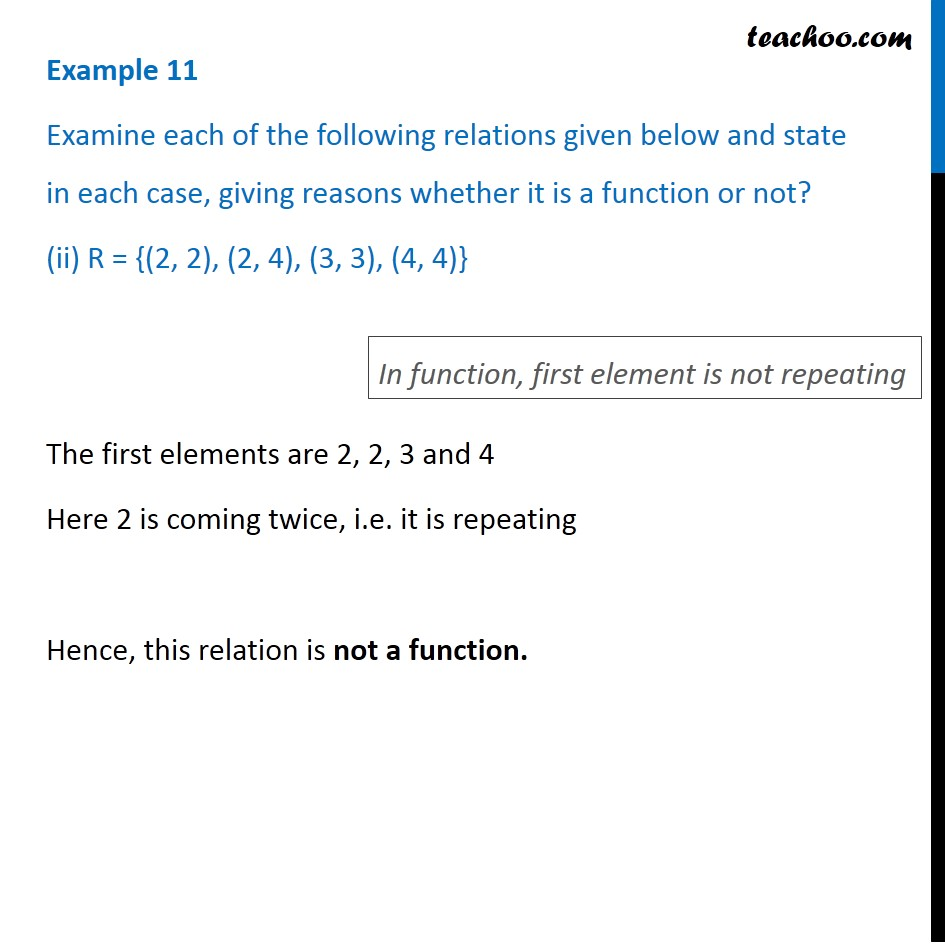1. Chapter 2 Class 11 Relations and Functions (Term 1)
2. Serial order wise
3. Examples

Transcript

Example 11 Examine each of the following relations given below and state in each case, giving reasons whether it is a function or not? (ii) R = {(2, 2), (2, 4), (3, 3), (4, 4)} The first elements are 2, 2, 3 and 4 Here 2 is coming twice, i.e. it is repeating Hence, this relation is not a function. In function, first element is not repeating